+关注继续查看

# 一、面向过程与面向对象

C语言作为一种面向过程的编程语言，注重解决问题的过程和步骤，通过函数和控制流程的设计来组织程序。

C++是基于面向对象的，关注的是对象，将一件事情拆分成不同的对象，靠对象之间的交互完成。## 面向过程分析：(C语言)

1.收集所需材料和工具：面包、黄油、火腿、生菜、刀子、砧板等。

2.将切好的面包放在砧板上。

3.使用刀子涂抹黄油在面包片上。

4.在其中一片面包上放上火腿和生菜。

5.将另一片面包盖在火腿和生菜上，使之成为一个完整的三明治。

6.可选：将整个三明治切成两半或四等份。

7.完成。

## 面向对象分析：(C++)

1.定义一个"三明治"类，它具有属性（面包、黄油、火腿、生菜）和方法（涂抹黄油、放置火腿和生菜、组装成三明治）。

2.创建一个"三明治"对象。

3.调用对象的方法，按照特定的顺序执行：

4.可选：调用切割的方法，将整个三明治切成两半或四等份。

5.完成。

# 二、类

## 2.1 类的介绍

C语言中: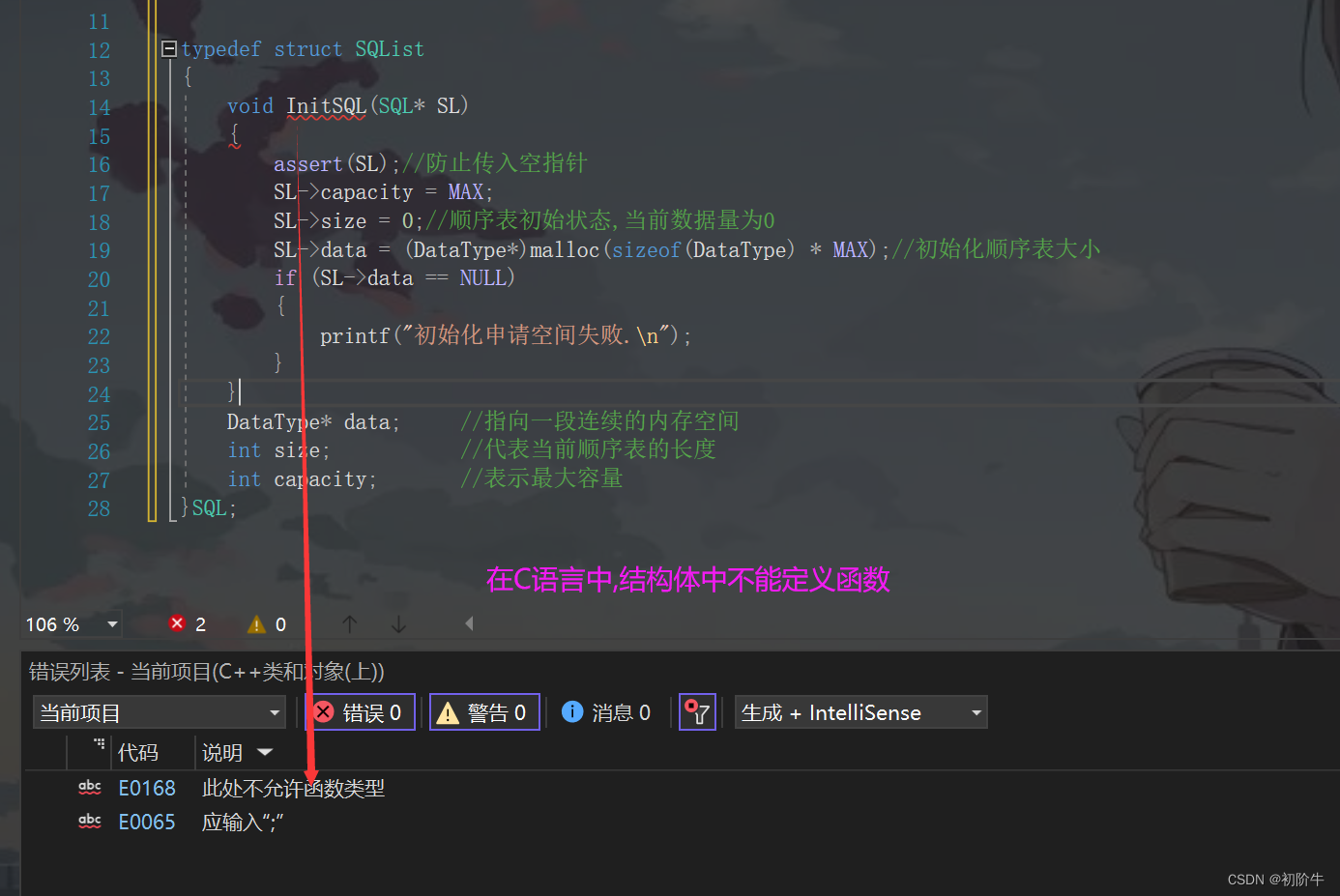C++中: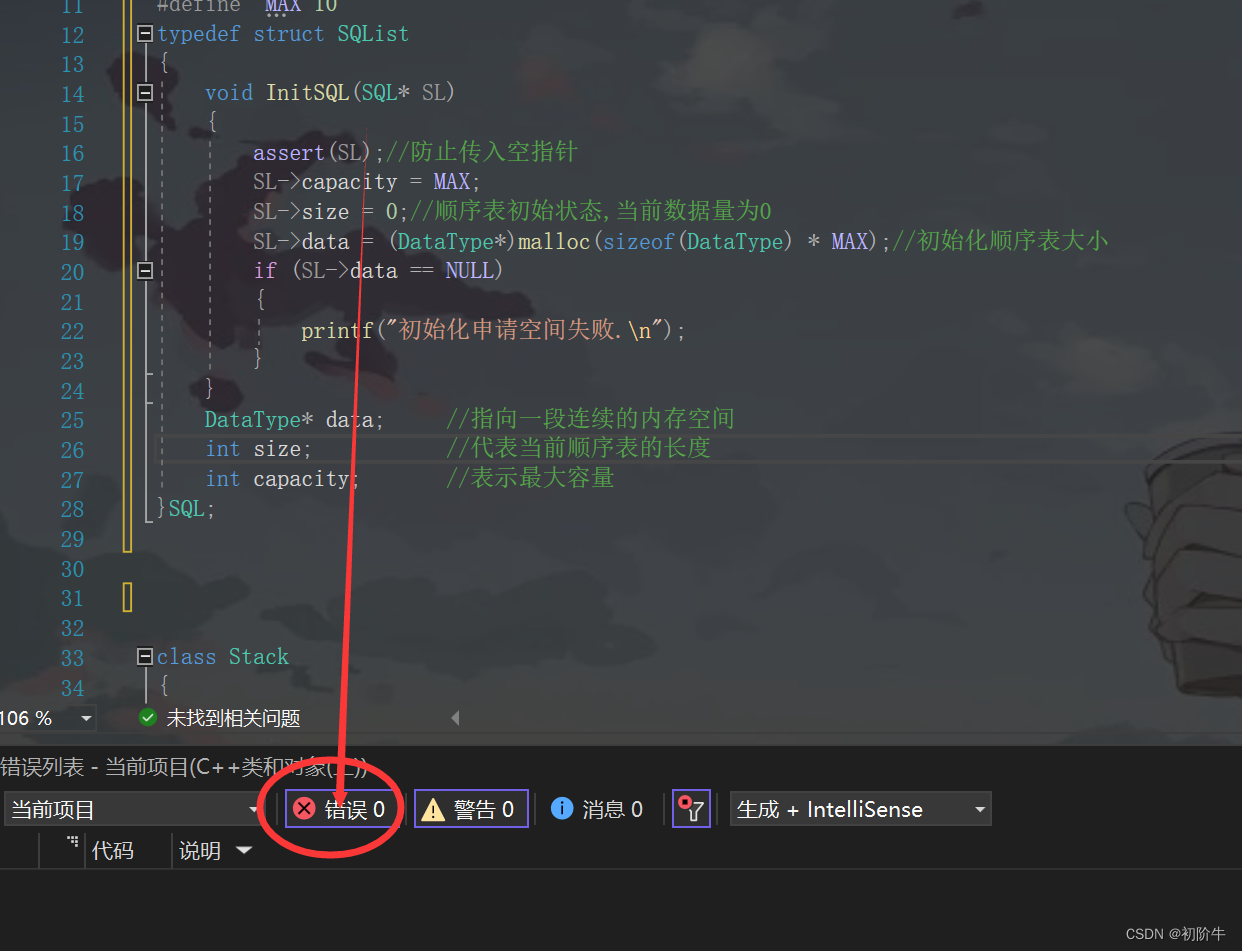## 2.2 类的定义方式

### (1)声明和定义全部放在类体中.


#include <iostream>

using namespace std;//在工程代码中不建议展开可能会产生命名冲突

class Person {
public:
// 构造函数(后面会讲,这里按普通成员函数理解)
Person(char* n, int a) {
name = n;
age = a;
}

// 成员函数
void introduce() {
cout << "欢迎来到CSDN!\n我是" << name << ",我的年龄是" << age << "岁" << endl;
}
private:
//成员变量
char* name;
int age;
};

int main() {
char name[] = "初阶牛";
Person person(name, 18);
person.introduce();

return 0;
}


欢迎来到CSDN!



### (2)类的声明和"成员函数"分离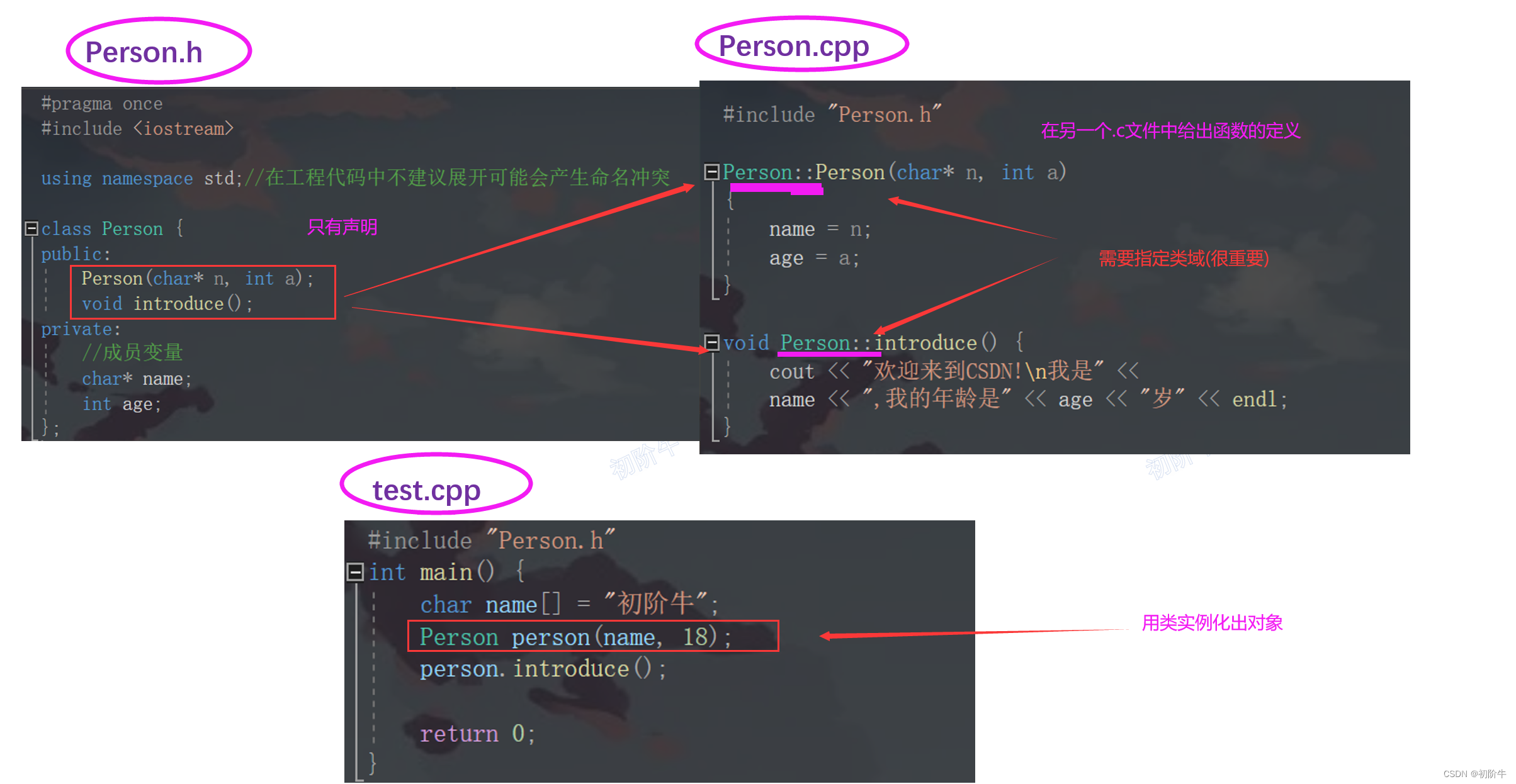## 2.3 类的访问限定符

10公共访问（public）：使用public关键字来指定。公共成员可以从任何地方访问，包括外部代码和其他类。公共成员在整个程序中可见。

2.私有访问（private）：使用private关键字来指定。私有成员只能在声明它们的类内部访问。其他任何外部代码或其他类都无法直接访问私有成员，包括子类。

3.受保护访问（protected）：使用protected关键字来指定。受保护成员只能在声明它们的类内部访问以及该类的子类中访问。外部代码无法直接访问受保护成员。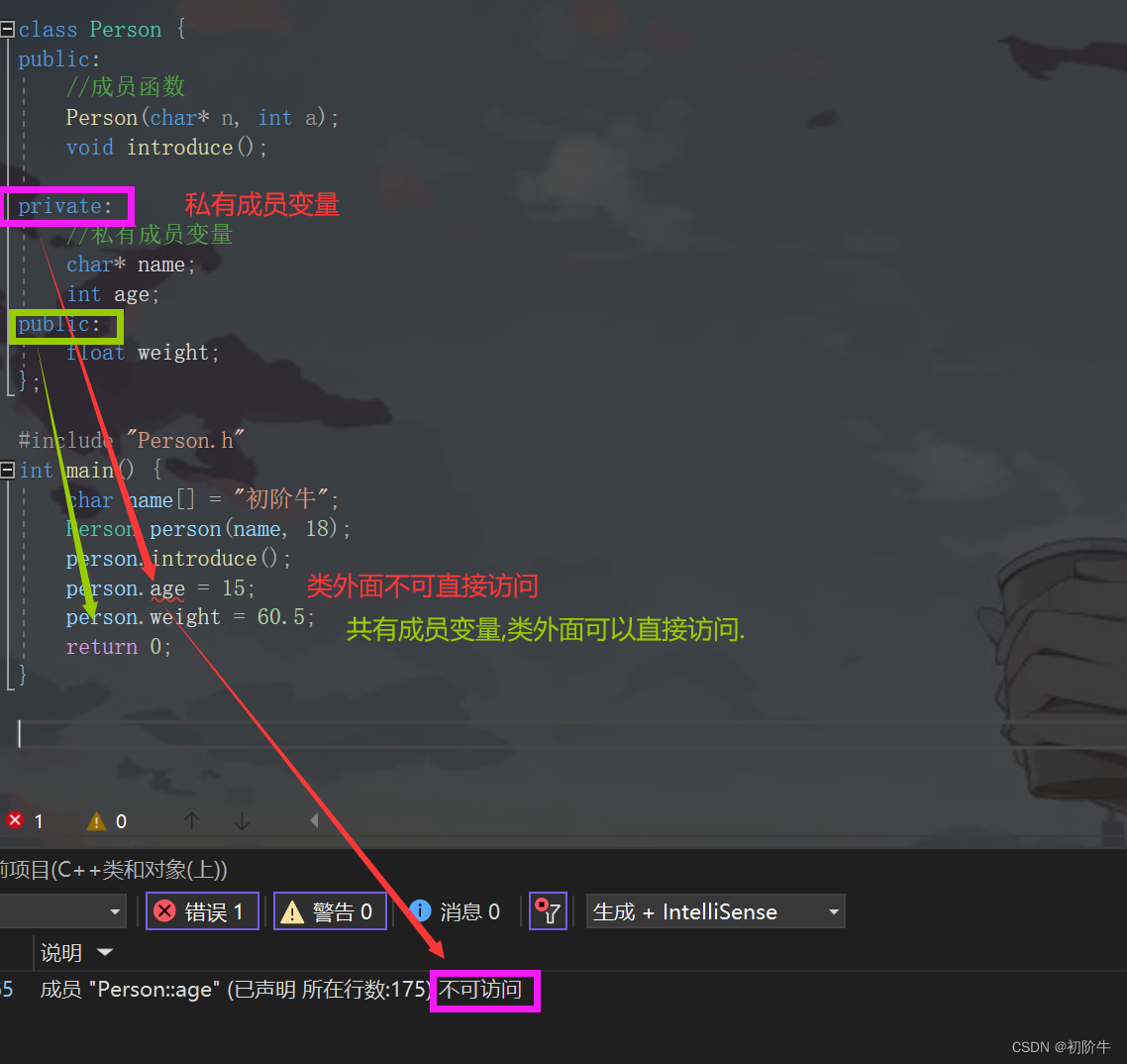1.C++中class(类)的默认访问级别是私有访问（private）。类的成员将默认为私有成员，只能在类内部访问。

2.struct(结构体)为public(因为struct要兼容C语言),在C语言中,外部可以访问结构体中的成员变量.

## 2.4 封装的介绍# 三、“类” 与 “对象” 之间的关系

class Person {
public:
//成员函数
Person(char* n, int a);
void introduce();

private:
//私有成员变量
char* name;
int age;
public:
float weight;
};

void test2()
{
//报错
Person.weight = 60.5;//报错,类只是声明,并没有申请空间,不能用于存放数据

//正确写法
char name[] = "初阶牛";
Person cjn(name, 18);//通过类实例化出 cjn这个对象.
cjn.introduce();
cjn.weight = 60.5;//实例化出来的对象是有空间的,可以存储数据

}


1.类是对对象进行描述的，是一个模型一样的东西，限定了类有哪些成员，定义出一个类并没有分配实际的内存空间来存储它；

2.一个类可以实例化出多个对象，实例化出的对象 占用实际的物理空间，存储类成员变量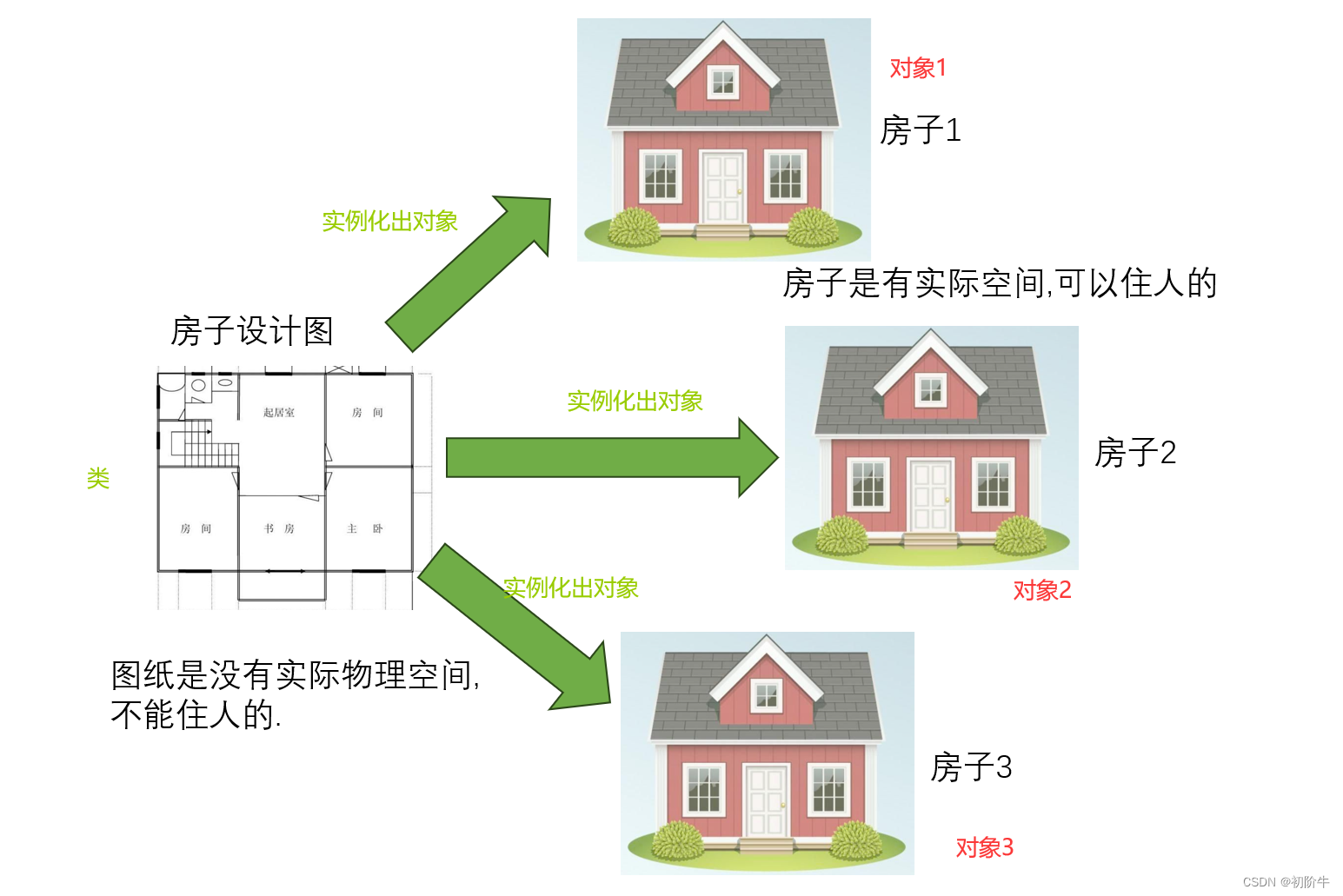## 3.1 类的大小计算

#include <iostream>

using std::cout;
using std::cin;
using std::endl;

class People
{
public:
void Print()
{
int a = 0;
double b= 1.2;
cout << _name << endl;
}
private:
char _name;
int _age;
};

int main()
{
cout << sizeof(People) << endl;
return 0;
}8

char _name占1个字节(偏移量为0),int _age占四个字节(4-7偏移量).共八个字节.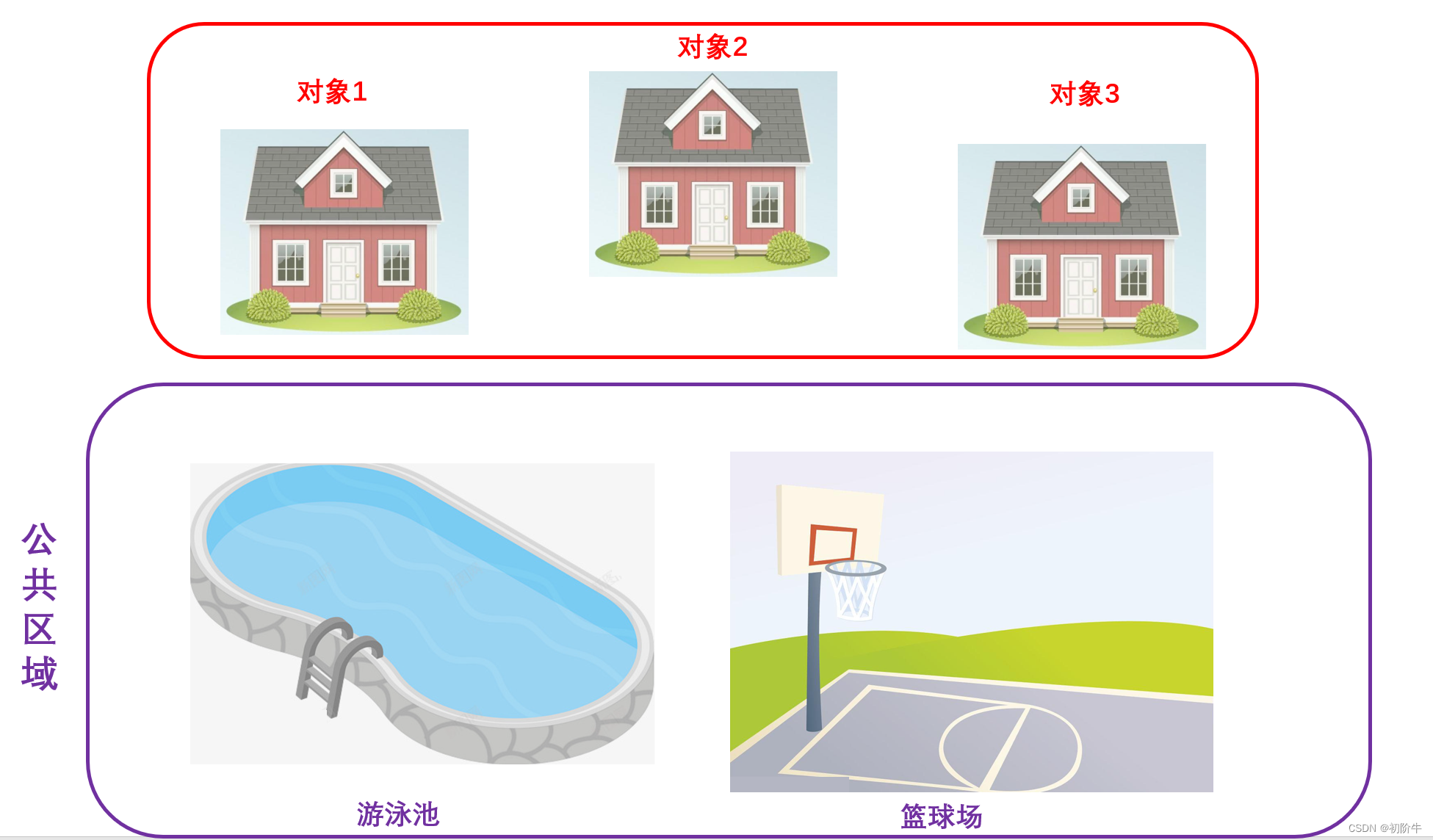// 只有成员函数的类
class A {
public:
void test() {}
};
//空类
class B

{};

int main()
{
cout << sizeof(A) << endl;
cout << sizeof(B) << endl;
return 0;
}1
1

## 3.2 this指针

#include <iostream>

using std::cout;
using std::cin;
using std::endl;

class Person {
public:
//成员函数
Person(char* n, int a)
{
_name = n;
_age = a;
}

void introduce()
{
cout << _name << endl;
}

private:
//私有成员变量
char* _name;
int _age;
};

int main()
{
char name1[] = "初阶牛";
char name2[] = "CSDN";
Person person1(name1, 18);
Person person2(name2, 18);
//这两个调用的是同一个函数吗?
person1.introduce();
person2.introduce();
return 0;
}


初阶牛
CSDN

    person1.introduce();
person2.introduce();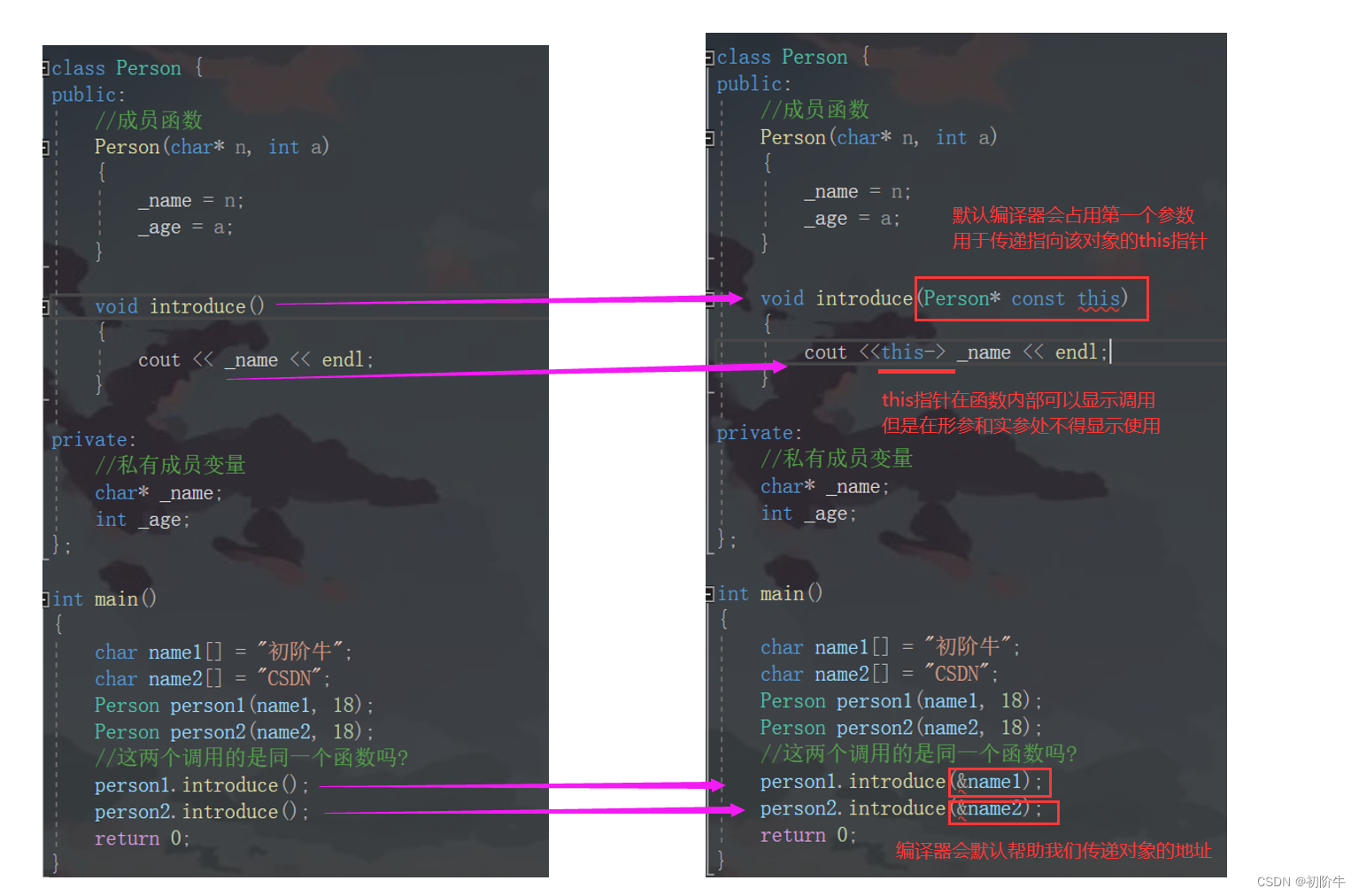## 3.3 深入理解this指针

A. 栈区

B. 堆区

C. 对象中

D. 常量区//代码1:
class A
{
public:
void Print()
{
cout << "HELLO CSDN" << endl;
}
private:
int _a;
};
int main()
{
A* p = nullptr;
p->Print();
return 0;
}

// 代码2:
class A
{
public:
void Print()
{
cout << _age << endl;
}
private:
int _age;
};
int main()
{
A* p = nullptr;
p->Print();
return 0;
}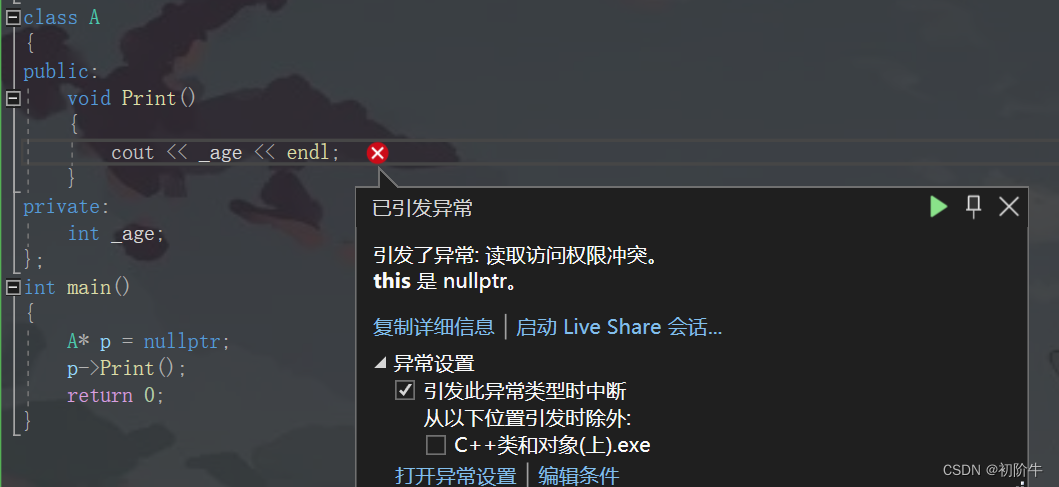# 四、C与C++对比

C语言数据和方法是分离的,给予C程序员很大的操作空间.这样也就使得对C程序员的要求很高.太自由了!

C++程序员受封装的保护,对于栈中的很多操作只能通过调用对应的接口实现,更好的约束了程序员的操作规范

## C实现栈:

//C语言版本
#include <stdio.h>
#include <assert.h>
#include <stdlib.h>
#include <stdbool.h>

typedef int DataType;
typedef struct Stack
{
DataType* array;
int capacity;
int size;
}Stack;
void StackInit(Stack* ps)
{
assert(ps);
ps->array = (DataType*)malloc(sizeof(DataType) * 3);
if (NULL == ps->array)
{
assert(0);
return;
}
ps->capacity = 3;
ps->size = 0;
}
void StackDestroy(Stack* ps)
{
assert(ps);
if (ps->array)
{
free(ps->array);
ps->array = NULL;
ps->capacity = 0;
ps->size = 0;
}
}
void CheckCapacity(Stack* ps)
{
if (ps->size == ps->capacity)
{
int newcapacity = ps->capacity * 2;
DataType* temp = (DataType*)realloc(ps->array,
newcapacity * sizeof(DataType));
if (temp == NULL)
{
perror("realloc申请空间失败!!!");
return;
}
ps->array = temp;
ps->capacity = newcapacity;
}
}
void StackPush(Stack* ps, DataType data)
{
assert(ps);
CheckCapacity(ps);
ps->array[ps->size] = data;
ps->size++;
}
int StackEmpty(Stack* ps)
{
assert(ps);
return 0 == ps->size;
}
void StackPop(Stack* ps)
{
if (StackEmpty(ps))
return;
ps->size--;
}
DataType StackTop(Stack* ps)
{
assert(!StackEmpty(ps));
return ps->array[ps->size - 1];
}
int StackSize(Stack* ps)
{
assert(ps);
return ps->size;
}
int main()
{
Stack s;
StackInit(&s);
StackPush(&s, 1);
StackPush(&s, 2);
StackPush(&s, 3);
StackPush(&s, 4);
printf("%d\n", StackTop(&s));
printf("%d\n", StackSize(&s));
StackPop(&s);
StackPop(&s);
printf("%d\n", StackTop(&s));
printf("%d\n", StackSize(&s));
StackDestroy(&s);
return 0;
}


## C++实现栈:

typedef int DataType;
class Stack
{
public:
void Init()
{
_array = (DataType*)malloc(sizeof(DataType) * 3);
if (NULL == _array)
{
perror("malloc申请空间失败!!!");
return;
}
_capacity = 3;
_size = 0;
}
void Push(DataType data)
{
CheckCapacity();
_array[_size] = data;
_size++;
}
void Pop()
{
if (Empty())
return;
_size--;
}
DataType Top() { return _array[_size - 1]; }
int Empty() { return 0 == _size; }
int Size() { return _size; }
void Destroy()
{
if (_array)
{
free(_array);
_array = NULL;
_capacity = 0;
_size = 0;
}
}
private:
void CheckCapacity()
{
if (_size == _capacity)
{
int newcapacity = _capacity * 2;
DataType* temp = (DataType*)realloc(_array, newcapacity *
sizeof(DataType));
if (temp == NULL)
{
perror("realloc申请空间失败!!!");
return;
}
_array = temp;
_capacity = newcapacity;
}
}
private:
DataType* _array;
int _capacity;
int _size;
};
int main()
{
Stack s;
s.Init();
s.Push(1);
s.Push(2);
s.Push(3);
s.Push(4);

printf("%d\n", s.Top());
printf("%d\n", s.Size());
s.Pop();
s.Pop();
printf("%d\n", s.Top());
printf("%d\n", s.Size());
s.Destroy();
return 0;
}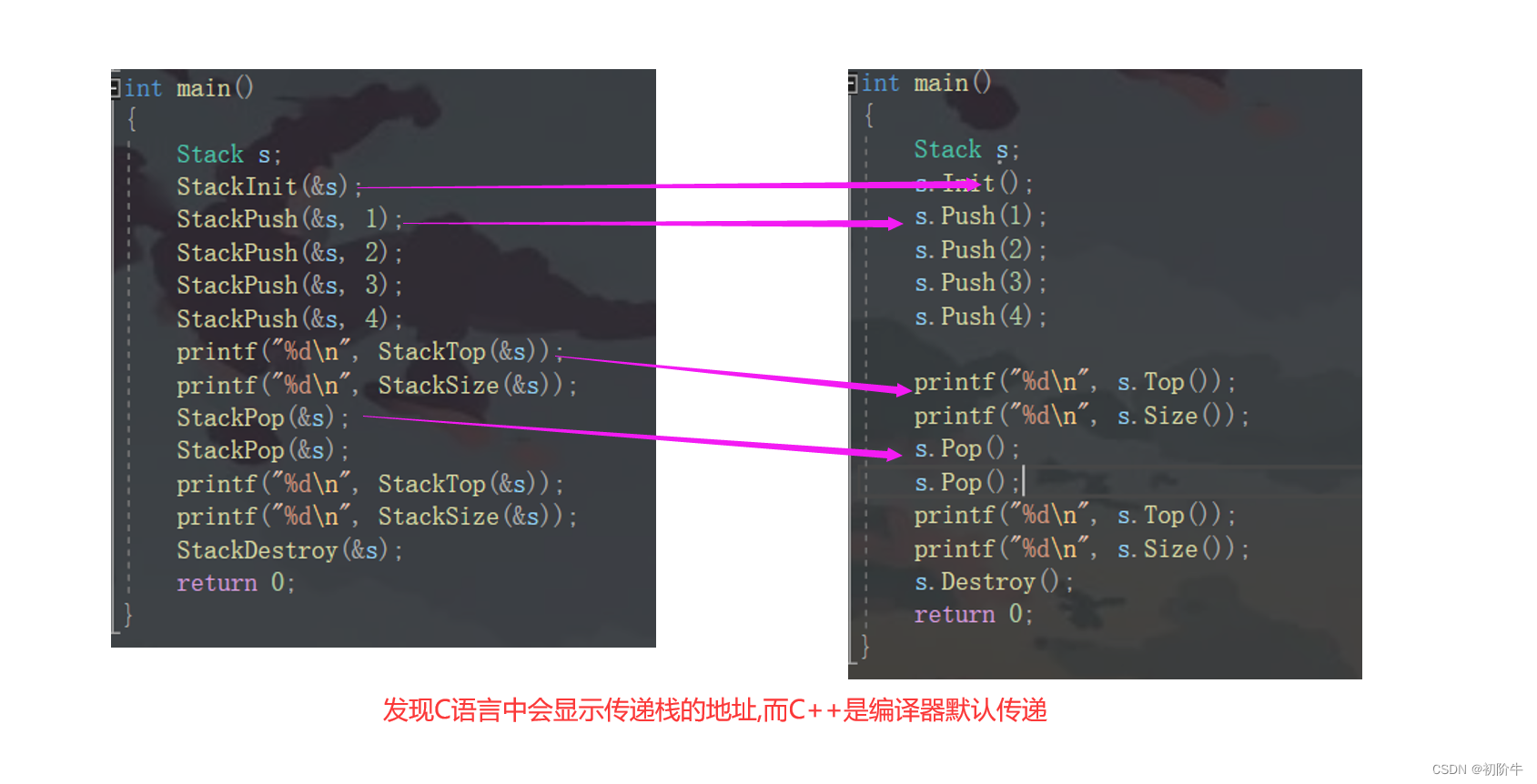|
3天前
|

c++拷贝对象时的优化问题

12 0
|
8天前
|
Kubernetes NoSQL 网络协议
C++中作为类实例的对象
C++中作为类实例的对象
11 0
|
8天前
|

13 0
|
8天前
|

7 0
|
8天前
|

C++类的对象占用多大的内存空间？
C++类的对象占用多大的内存空间？
12 0
|
8天前
|

CLI封装C++对象，供C#程序调用
CLI封装C++对象，供C#程序调用
12 0
|
25天前
|

【C++从0到王者】第七站：内存管理（520没有对象?那就new一个）
【C++从0到王者】第七站：内存管理（520没有对象?那就new一个）
14 0
|
26天前
|
Java 编译器 C++
C++冷知识：构造函数初始化时，为什么使用 : 而不是使用作用域内初始化对象？

14 0
|
29天前
|

C++ 对象生成：构造函数
C++ 对象生成：构造函数
17 0
|
1月前
|
C++
84 C++ - 内建函数对象
84 C++ - 内建函数对象
13 0